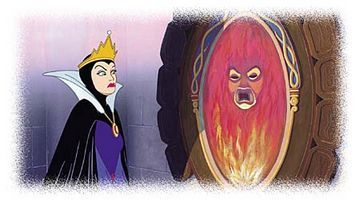# Mirror, mirror, on the wall, who's the fairest of them all?A positive integer $n$ is called fair if the sum of the factorial of its digits is divisible by itself. 145 is one such number : $1! + 4! + 5! = 145 \equiv 0 \mod (145)$ Find the sum of all the fair numbers less than $10^7$.

×